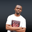Related Tags

conditionals
operators
php
communitycreator

# What are the conditional assignment operators in PHP?NDUKWE CHIDERA K.

### Introduction

Operators in PHP are signs and symbols which are used to indicate that a particular operation should be performed on an operand in an expression. Operands are the operation objects, or targets. An expression contains operands and operators arranged in a logical manner.

In PHP, there are numerous operators, which are grouped into the following categories:

• Logical operators

• String operators

• Array operators

• Arithmetic operators

• Assignment operators

• Comparison operators

• Increment/Decrement

• Conditional assignment operators

Some examples of these operators are:

• + Addition
• - Subtraction
• * Multiplication
• / Division
• % Modulus
• >
• <
• =
• ++
• -- and so much more.

### What are conditional assignment operators?

Conditional assignment operators, as the name implies, assign values to operands based on the outcome of a certain condition. If the condition is true, the value is assigned. If the condition is false, the value is not assigned.

There are two types of conditional assignment operators: tenary operators and null coalescing operators.

#### 1. Tenary operators ?:

##### Basic syntax
$y = expr1 ? expr2 : expr3;  Where expr1, expr2, and expr3 are either expressions or variables. From the syntax, the value of $y will be evaluated. This value of $y is evaluated to expr2 if expr1 = TRUE. The value of$y is expr3 if expr1 = FALSE.

#### 2. Null coalescing operators ??

The ?? operator was introduced in PHP 7 and is basically used to check for a NULL condition before assignment of values.

$z = varexp1 ?? varexp2;  Where varexp1and varexp2 are either expressions or variables. The value of $z is varexp1 if and only if varexp1 exists, and is not NULL. If varexp1 does not exist or is NULL, the value of $z is varexp2. ### Code Let’s use some code snippets to paint a more vivid description of these operators. <?php /*** Ternary operator ***/$expr1 = 7 > 10;
$expr2 = "New y value if expr1 is true";$expr3 = "New y value if expr1 is false";

//$y takes the value of$expr3 because $expr1 is false$y = $expr1 ?$expr2:$expr3; echo$y;

/**** Null coalescing operator ****/
echo "\n";
//set varexp1 value to null
$varexp1 = null; // set varexp2 value to string.$varexp2 = "new z value as varexp1 is null";

$z =$varexp1 ?? $varexp2; echo$z;

?>

### Explanation

In the code above, the new value of $y and $z is set based on the evaluation result of expr1 and varexp1, respectively.

RELATED TAGS

conditionals
operators
php
communitycreator

CONTRIBUTORNDUKWE CHIDERA K.
RELATED COURSES

View all Courses

Keep Exploring

Learn in-demand tech skills in half the time